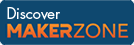Cody

# Problem 640. Getting logical indexes

Created by Doug Hull in Community

This is a basic MATLAB operation. It is for instructional purposes.

---

Logical indexing works like this.

```thresh = 4;
vec    = [1 2 3 4 5 6 7 8];
```
```vi     = (vec > thresh)
```
```vi =
```
`       0     0     0     0     1     1     1     1`

Once you have this TRUE FALSE vector (I call it vi: Valid Indices)

It can be used to get the values out:

```big = vec(vi)
```
```big =
```
`       5     6     7     8`

Given a vector, vec, and a value, v, return a binary vector that represents the indices where vector, vec, is equal to scalar, v.

Note, this works just as well with scalars and matrices.

----

To get the indices where this comparison is true, see this Cody problem.

### Solution Stats

58.84% Correct | 41.16% Incorrect
Last solution submitted on Mar 25, 2019

#### TagsMATLAB and Simulink resources for Arduino, LEGO, and Raspberry Pi